Thank you for visiting nature.com. You are using a browser version with limited support for CSS. To obtain the best experience, we recommend you use a more up to date browser (or turn off compatibility mode in Internet Explorer). In the meantime, to ensure continued support, we are displaying the site without styles and JavaScript.

# Inelastic ponderomotive scattering of electrons at a high-intensity optical travelling wave in vacuum

## Abstract

In the early days of quantum mechanics Kapitza and Dirac predicted that matter waves would scatter off the optical intensity grating formed by two counter-propagating light waves1. This interaction, driven by the ponderomotive potential of the optical standing wave, was both studied theoretically and demonstrated experimentally for atoms2 and electrons3,4,5. In the original version of the experiment1,5, only the transverse momentum of particles was varied, but their energy and longitudinal momentum remained unchanged after the interaction. Here, we report on the generalization of the Kapitza–Dirac effect. We demonstrate that the energy of sub-relativistic electrons is strongly modulated on the few-femtosecond timescale via the interaction with a travelling wave created in vacuum by two colliding laser pulses at different frequencies. This effect extends the possibilities of temporal control of freely propagating particles with coherent light and can serve the attosecond ballistic bunching of electrons6, or for the acceleration of neutral atoms or molecules by light.

## Main

Depending on the scattering regime, the interaction between electrons and the ponderomotive potential of an optical standing wave can be described both quantum mechanically (Kapitza–Dirac effect7,8,9) or classically8,10. In the quantum picture, the matter wave coherently diffracts on the periodic potential of the two colliding optical waves with wavevectors k and −k and identical frequency ω, leading to observation of a series of diffraction peaks separated by two photon recoils 2 k (Raman–Nath regime5,7) or an individual diffraction peak (Bragg regime8,11). From the point of view of energy and momentum conservation, a diffracted particle simultaneously absorbs a photon from the first wave and emits a photon to the second wave via stimulated Compton scattering. The strength of the interaction is proportional to the light intensity (density of photons) of the optical standing wave.

From the classical perspective describing an incoherent scattering regime4,8,10, the periodic ponderomotive potential of the optical standing wave Up = e2 |E0|2/(m0ω2)cos2(k r), where e is electron charge, m0 is electron mass, r is position vector and E0 is the field amplitude of each wave, leads to scattering of electrons due to the spatial dependence of the transverse ponderomotive force Fp = −Up. Also more general cases of inelastic scattering of electrons by two-colour fields have been proposed12,13,14,15, and ponderomotive ballistic bunching of electron beams was theoretically considered6,16,17. However, experiments along these lines have not been realized hitherto.

In this paper we experimentally demonstrate a strong modulation of energy and longitudinal momentum of electrons (momentum component in the direction of electron propagation) in vacuum by using two pulsed laser beams at different frequencies ω1 and ω2 intersecting with a pulsed electron beam at non-zero angles of incidence α and β (Fig. 1a–c). These laser fields create an optical travelling wave propagating parallel to the electron beam with a group velocity vg = (ω1ω2)c/(ω1 cosαω2 cosβ) (where c is the speed of light), which can be synchronized with the electron initial velocity vi (see Supplementary Information for details). The electrons thus inelastically scatter at the travelling wave, leading to a broadening of their energy spectra. The ponderomotive potential an electron experiences in this case has the form16: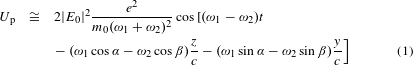where z is the electron propagation direction, y is the coordinate perpendicular to z in the plane defined by the two laser beams, and t is time. In the electron’s rest frame, a standing optical wave is formed. Therefore, the electron experiences a constant phase with respect to the light intensity modulation and is pushed out of the high-intensity regions by the ponderomotive force (shown schematically in Fig. 1e). An arbitrary choice of incidence angles α and β leads to an angular tilt of the travelling wave with respect to z (term −(ω1 sinαω2 sinβ)y/c in equation (1)), and consequently to the modulation of the electron’s transverse momentum. Here we show that for a particular combination of light frequencies and incidence angles of the two laser beams, only the longitudinal component of the electronmomentum changes.Figure 1: Layout of the experiment demonstrating the inelastic ponderomotive scattering of electrons at a high-intensity optical travelling wave in vacuum.

The laser pulses are obtained by optical parametric generation, where two photons with energies ω1 > ω2 and ω2 are produced from the incident photon with energy ω = ω1 + ω2. Diagrams describing the individual scattering events of electrons in vacuum are shown in Fig. 1a–c. While Fig. 1a shows the situation before, Fig. 1b shows the system after the stimulated Compton scattering process where a photon with higher energy is absorbed by an electron while a photon with lower energy is emitted. The incidence angles α and β of the laser beams are selected in such a way that the electron momentum change Δ k is parallel to its initial momentum kin, leading to zero transverse momentum change. The second possible process (Fig. 1c) leads to a decrease of electron energy/momentum.

The relativistic energy and momentum conservation laws for the case of zero transverse momentum transfer Δ k = 0 for the system of an electron and two photons with different energies can be written as: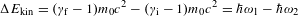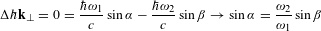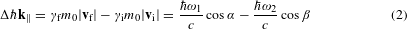Here Δ kǁ is the longitudinal momentum change of the electron, ΔEkin is the change of the electron kinetic energy and γf, i = (1 − |vf, i|2/c2)−1/2 are the relativistic Lorentz factors corresponding to the final and initial electron velocities vf and vi, respectively. Because the frequencies of the two interacting photons are not independent in our experiment, due to the parametric generation process, the set of equations (2) leads to a formula defining the angles α and β as a function of the initial electron velocity vi and frequency ω1: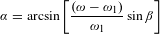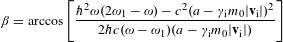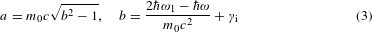This equation shows that the experimental parameters for purely longitudinal momentum transfer, namely the values of α, β, ω1 and ω2, can be adapted to any initial electron velocity 0 ≤ |vi| < c (see Supplementary Fig. 1 for details).

The experimental demonstration of the inelastic ponderomotive scattering of electrons at a high-intensity optical travelling wave is carried out in the vacuum chamber of a modified scanning electron microscope (SEM), which serves as a pulsed source of electrons that are photoemitted from the SEM cathode (Schottky tip) by an ultraviolet laser pulse. After acceleration by electrostatic fields to the final kinetic energy of Ekin = 29 keV, electrons are focused to the interaction region. Here they experience the optical fields of the two femtosecond laser pulses at wavelengths of λ1 = 2πc/ω1 = 1,356 nm (ω1 = 0.91 eV) and λ2 = 1,958 nm (ω2 = 0.63 eV). The two beams intersect with the electron beam under angles α = (41 ± 2)° and β = (107 ± 2)° obtained from equation (3). Electron spectra after the interaction are measured by an electromagnetic spectrometer and a micro-channel plate (MCP) detector (Fig. 1d). For a more detailed description of the experimental set-up and its characterization, see Methods.

The measured electron spectra in the presence (red curve) and absence (grey curve) of the optical travelling wave are plotted in Fig. 2a, in comparison with numerical calculations (dashed curve). With laser pulses in both beams having equal pulse energies of Ep = 85 μJ, leading to a peak optical intensity of Ip = 3 × 1015 W cm−2, we observe broad shoulders in the spectrum. The large energy modulation of more than 10 keV is a consequence of the high-intensity gradient caused by the small period λg = 2πc/(ω1 cosαω2 cosβ) = 1.41 μm of the travelling wave.Figure 2: Measurements of electron spectra after the inelastic ponderomotive scattering at a high-intensity optical travelling wave in vacuum.

Due to the periodicity of the scattering potential in the longitudinal direction of the electron wavepacket propagation, coherent interference peaks, separated by the difference between the energies of the two photons participating in the scattering ΔE = ω1ω2, are expected in the electron spectra as a consequence of the quantum interference between electron matter waves scattered by subsequent periods of the travelling wave. This is analogous to the classical Kapitza–Dirac experiment in the diffraction regime5, with the roles of the transverse and the longitudinal directions exchanged. To reach the coherent regime of the interaction, the longitudinal coherence length of the electron beam has to be significantly longer than λg. This is, however, not the case in our experiment, where the coherence length can be estimated to be ξǁ = 2.35viEkin, in 1.8 μm (ref. 18), where δEkin, in = 0.5 eV is the expected initial energy spread (full-width at half-maximum, FWHM) of the electron beam.

The maximum observed energy modulation of the electrons corresponds to the simultaneous absorption and emission of more than 104 photons by a single electron. In this high-intensity regime, where the scattering rate exceeds the optical frequency of the driving light4, a depletion of the electron population around the initial energy and the appearance of two broad incoherent rainbow peaks are expected in the electron spectra, similar to the high-intensity Kapitza–Dirac effect4. However, the shape of the measured spectra is further influenced by the fact that the electron pulse duration τe, FWHM used in the experiment is longer than the durations of the laser pulses τ1, FWHM, τ2, FWHM, generating the optical travelling wave (see Methods for details). The non-ideal temporal overlap leads to a broad distribution of interaction strengths experienced by the electrons. The comparison between calculated electron spectra for the two cases τe, FWHM τ1, FWHM, τ2, FWHM and τe, FWHM τ1, FWHM, τ2, FWHM is shown in Supplementary Fig. 2.

Due to the incoherent scattering nature of the observed effect, the interaction can be described classically as a scattering of point-like particles at the travelling optical wave. Each calculated spectrum in Fig. 2a, b is therefore obtained by a Monte-Carlo simulation of the interaction between a set of particles and the two laser fields. The interaction is modelled by a numerical integration of the classical relativistic equation of motion with the Lorentz force (see Methods for details).

For further characterization, we measure electron spectra as a function of the energy of the two driving laser pulses in the pulse energy range 19–128 μJ (solid curves in Fig. 2b). Again, numerical simulations fit the data very well. The dependence of the induced energy spread δE as a function of pulse energy is shown in Fig. 2c. The spectrum with the maximum observed energy spread of 19.6 keV is obtained with a peak intensity of Ip = 5 × 1015 W cm−2, corresponding to a normalized field amplitude a0 = eE0/(m0) = 0.1 1. The experiment presented here thus occurs in the sub-relativistic field regime (for details see Methods).

To characterize the transverse momentum transfer and prove that it is negligible, we measure the two-dimensional (2D) spatial distribution of the electrons on the MCP detector after the spectrometer (Fig. 2e). A modulation of the transverse momentum of electrons would lead to their deflection in y and would appear here as a spread along the ydet axis (xdet and ydet being the Cartesian coordinates in the detector plane, see Fig. 1d). The observed deflection angles of electrons are below the angular resolution of the set-up (δθdef 20 mrad), confirming that the transverse momentum transfer is negligibly small compared to the longitudinal momentum change (see Methods).

For applications in particle acceleration by laser fields19,20, an important property of the demonstrated inelastic scattering is the peak value of the energy gain per unit length, the acceleration gradient. In this proof-of-concept experiment we reach Gp = dEkin/dz = 2.2 ± 0.2 GeV m−1 (Fig. 2d). Albeit obtained in a second-order ponderomotive process, this gradient is already much higher than typical values reached in state-of-the-art radiofrequency accelerators (50 MeV m−1). In addition it is almost on par with the Gp = 3 GeV m−1 obtained using vacuum acceleration of electrons by the longitudinal field of radially polarized few-cycle pulses in the relativistic field regime (a0 = 5) (ref. 21). The high efficiency makes the ponderomotive interaction between electrons and an optical travelling wave interesting for applications in various particle acceleration schemes, such as in laser-wakefield or dielectric laser acceleration, where it could serve for initial pre-acceleration. Furthermore, because of the independence of the ponderomotive force on the sign of the charge, spacetime compression of plasmas is possible.

As an important consequence of the periodic sinusoidal modulation of the longitudinal electron momentum on the femtosecond timescale, an attosecond bunch train is generated due to a rotation of the electron distribution in longitudinal phase space during the ballistic propagation (propagation without any external forces) after the interaction6,16,17. This opens a way to reach sub-optical cycle—that is, attosecond (1 as = 10−18 s)—temporal resolution in ultrafast electron diffraction and microscopy experiments, or to control the electron injection in novel photonics-based accelerators on attosecond timescales22,23. Numerical simulation results of the ballistic bunching of electrons after their interaction with the optical travelling wave are shown in Fig. 3 for the two central periods of the temporal intensity envelope. The calculation is performed with the experimental parameters used in this study, namely pulse energies of Ep = 19 μJ (the lowest spectrum in Fig. 2b) and an initial electron energy spread of δEkin, in = 0.5 eV.Figure 3: Attosecond ballistic bunching of electrons after the interaction with the optical travelling wave.

The temporal focus occurs already at a propagation distance of 11 μm after the centre of the interaction defined by the point of the intersection of the three beams (the electron beam and the two laser beams) used in the experiment. This corresponds to a propagation time of only 110 fs. The resulting minimum temporal duration of an individual electron bunch in the train is simulated to be τFWHM = 210 ± 10 as, and 30% of all electrons spread initially over one period of the travelling wave (Tg = 2π/(ω1ω2) = 14.7 fs) are confined within a temporal window of 300 as in the temporal focus. This number can be further improved by a multistage compression scheme, where, in the first stage, the electron packet will be pre-bunched to a duration of a few femtoseconds by the interaction with a ponderomotive potential of a higher-order Laguerre–Gaussian spatial mode of a focused laser beam16. After the first compression stage, the electrons will be injected to a fraction of the period of an optical travelling wave, where the ponderomotive potential can be considered as parabolic in the longitudinal direction. Such a double-stage compression scheme will allow generation of an isolated electron attosecond pulse.

For bunching over macroscopic distances of 100 μm to 5 mm, which would allow temporal compression of the electrons while propagating to a sample under study in ultrafast electron diffraction experiments24,25, laser pulses with a pulse energy of just 100 nJ to 1 μJ are required. These are readily achievable even for megahertz repetition rate laser systems. Interestingly, the attosecond bunch train can be synchronized with an optical pulse produced by difference frequency mixing of the two waves used for the interaction as the optical period of such a pulse matches the time period of the optical travelling wave. In addition, and even without carrier-envelope phase stable laser pulses, a passive phase stability is obtained between the difference frequency wave and the optical travelling wave.

The demonstration of the inelastic ponderomotive scattering of electrons at an optical travelling wave presented here further opens the possibilities to study the quantum nature of nonlinear two-photon inelastic scattering/diffraction processes in vacuum in the energy domain. Similar to single-photon transitions induced by optical near fields26,27,28, two-photon quantum transitions can be observed employing a high-resolution electron energy loss spectrometer (EELS) in a transmission electron microscope-based set-up. Likewise Ramsey-type interferometry experiments29,30 are possible via two subsequent interactions. This technique can further serve for energy modulation and bunching of propagating atoms or molecules, where the interaction strength can be even further enhanced using near-resonant interactions2. Last, by controlling the polarization state of the two pulses, namely using a combination of linearly and circularly polarized light, energy-resolved studies of electron spin flipping or spin polarization-dependent splitting in high-intensity laser fields might be performed using the presented scheme31,32,33.

## Methods

### Laser pulses.

We use a Ti:sapphire regenerative amplifier (repetition rate frep = 1 kHz, pulse duration τFWHM = 90 fs, central wavelength λ = 800 nm, pulse energy Ep = 7 mJ) to serve as a pump for an optical parametric amplifier (OPA). Here the three laser beams for the experiment are generated. The optical travelling wave is formed by the signal and idler pulses from the OPA with linear polarizations perpendicular to the plane of incidence. The incidence angles of the two beams with respect to the electron beam are α = (41 ± 2)° and β = (107 ± 2)°. The spectrum of the two pulses is measured by an infrared spectrometer (see Supplementary Fig. 3a). Here the central wavelengths of λ1 = 1,356 nm (ν1 = ω1/2π = 221.1 THz), λ2 = 1,958 nm (ν2 = 153.1 THz) and the spectral bandwidths of δλ1, FWHM = 47 nm (δν1 = 7.66 THz), δλ2, FWHM = 86 nm (δν2 = 6.72 THz) are obtained. The time–bandwidth product is measured for a particular OPA output wavelength by frequency-resolved optical gating to be τΔν = 0.38 (see Supplementary Fig. 3b). The pulse durations τ1, FWHM = 49 ± 5 fs and τ2, FWHM = 56 ± 6 fs are calculated from the spectral widths using the measured value of τΔν. The laser pulses are delivered to the SEM vacuum chamber and focused by two aspherical lenses with the same focal distance f = 25 mm. The transverse intensity profile of both laser beams is measured by an imaging set-up with a charge-coupled device (CCD) camera utilizing the two-photon absorption process. Here the 1/e2 radii of w0,1 = 10 ± 0.5 μm and w0,2 = 11.8 ± 0.6 μm are obtained (see Supplementary Fig. 3c, d). The angles of incidence of the two laser beams with respect to the electron propagation direction are measured in the vacuum SEM chamber. The spatio-temporal overlap of the two pulsed beams is reached by monitoring an optical four-wave mixing signal from a thin film of Al2O3, which is removed during the measurements. The ultraviolet (UV) pulse at a wavelength of λUV = 251 nm, which serves for photoemission of electrons in the SEM electron gun, is generated via sum-frequency mixing between a part of the signal beam from the OPA and the basic frequency beam from the amplifier, and subsequent second harmonic generation. The UV pulse is focused to the Schottky tip in the SEM electron gun by an UV achromatic lens with a focal length of f = 15 cm with polarization parallel to the tip axis. The relative timing of all three pulses is controlled using two independent optical delay lines (see the detailed layout of the experimental set-up in Supplementary Fig. 4).

The normalized field amplitude a0 is typically used to compare the strength of the interaction between laser fields and charged particles. In the relativistic field regime (a0 1), the field of the laser is strong enough to accelerate the particle close to the speed of light c during one optical cycle. In the opposite case (a0 1, this study), the change in the electron’s velocity during one optical cycle is small in comparison with c, and electrons do not reach relativistic energies (see the simulated energy increase of an accelerated electron during the interaction in Fig. 2d).

### Electron beam.

The electrons are photoemitted by a single-photon process from a Schottky-type cathode of the SEM using side-illumination. The initial electron energy in the experiment is Ekin = 29 keV. After the electron beam is focused by an objective lens, its transverse size at the interaction point is measured by the knife-edge technique (see Supplementary Fig. 5a) to be w = 3.6 ± 0.5 μm (1/e2 radius). The objective lens aperture is removed from the SEM column during the experiments to increase the electron beam current. The electron bunch duration τe, FWHM = 730 ± 30 fs is measured by acquiring the post-interaction electron spectra as a function of the time delay between the UV pulse and the two infrared pulses (see Supplementary Fig. 5b, c).

The transverse momentum of the electrons after the interaction in the plane of incidence of the two laser pulses (yz plane) is characterized in Fig. 2e by acquiring the 2D image of the electron distribution on the MCP detector. Here any deflection of electrons due to the interaction with the optical travelling wave would lead to spread/tilt of the electron distribution in the y direction, corresponding to ydet coordinate in the detector plane. The observed maximum deflection angle of electrons is below the angular resolution of the set-up (20 mrad). This is limited by defocusing of electrons in the ydet direction by the edge fields of the magnetic spectrometer (while the electrons are dispersed and focused in xdet) and the electron beam divergence angle. Negligible observed deflection of interacting electrons agrees with numerical simulations, where we obtain a maximum deflection angle of θdef 5 mrad (see Supplementary Fig. 6). This is caused by the non-zero width of the angular spectrum of the Gaussian beam plane wave representation in the focus34, the finite bandwidth of the laser pulses and the fact that the experimental conditions (angles of incidence α and β, laser frequencies ω1 and ω2) based on equation (3) are accurate only for electrons at the initial kinetic energy, while the energy of electrons in the experiment is significantly modulated already during the interaction.

### Detection set-up.

Electrons are dispersed by an Elbek-type electromagnetic spectrometer35 and detected by a Chevron-type MCP detector. The image of the MCP phosphor screen is acquired by a CCD camera. Each spectrum is obtained by integration of the above-threshold signal from 5,000 images with an exposure time of 0.1 s. The measured spectra are corrected by the detection efficiency curve of the MCP in the used energy range. The detection set-up is calibrated via the single-electron counting mode to obtain the number of electrons in each bin for the calculation of the shot noise of the measured electron current. The signal-to-noise ratio of the normal statistical distribution, where n is the detected number of electrons per energy bin, is used for determination of the experimental errors shown in Fig. 2a, b. The dispersion curve of the magnetic spectrometer is calculated to be close to linear for energies Ekin = 20–80 keV. The spectrometer and detection set-up performance (dispersion curve, resolution) is verified by a calibration procedure in the range 20–30 kV by adjusting the accelerating voltage of the SEM. The calibration curve is fitted by a parabola and extrapolated to higher energies. In the experimental spectra, the peak at the electron initial energy is caused by the fact that only 10% of electrons interact with the laser fields due to the different temporal durations of electron and laser pulses. The measured spectra have a characteristic shape with sharp cut-offs, after which the electron count rate decreases approximately linearly with energy. The energy spread δEkin is determined by fitting the high- and low-energy tails of each spectrum with a linear function f(x) = a ± bx. The intersection of the fitted line with the energy axis is considered to be the upper/lower edge (Ekin, upper, Ekin, lower) of the particular spectrum and δEkin = Ekin, upperEkin, lower.

### Simulations.

Simulations are performed by numerical integration of the relativistic equation of motion with the Lorentz force d/dt(γm0v) = q(E + v × B) by a fifth-order Runge–Kutta algorithm. As a result, the time evolution of the electron position r(t) and the velocity v(t) are obtained for each particle. The electric and magnetic fields of each pulsed laser beam are considered of Gaussian spatial and temporal mode, in the paraxial approximation: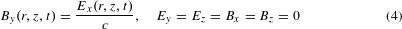where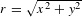, k = 2π/λ is the wavevector,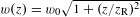the local beam radius, w0 the 1/e2 radius of the beam waist, R(z) = z[1 + (zR/z)2] the local radius of curvature of the phase front, φ(z) = arctan(z/zR) the Gouy phase and zR = πw02/λ the Rayleigh length of the beam. Standard rotation transformations are applied to describe the pulses incident under the angles α and β in the laboratory coordinate frame. Each electron energy spectrum is calculated in a Monte-Carlo simulation using 106 electrons with a Gaussian distribution in the transverse and longitudinal planes. The final spectra plotted in Fig. 2a, b result from a convolution of the calculated spectra with the response function of the detection set-up (grey curve in Fig. 2a). The initial electron energy spread is assumed to be δEkin = 0.5 eV (FWHM). Because the experiment is carried out in the regime of <1 electron/bunch, space charge forces are neglected in the simulations. In all simulations, the measured values of the transverse spot sizes and temporal durations of both laser pulses and the electron bunch are used.

### Data availability.

The data that support the plots within this paper and other findings of this study are available from the corresponding author upon reasonable request.

Publisher’s note: Springer Nature remains neutral with regard to jurisdictional claims in published maps and institutional affiliations.

## References

1. Kapitza, P. L. & Dirac, P. A. M. The reflection of electrons from standing light waves. Proc. Camb. Phil. Soc. 29, 297–300 (1933).

2. Gould, P. L., Ruff, G. A. & Pritchard, D. E. Diffraction of atoms by light: the near-resonant Kapitza–Dirac effect. Phys. Rev. Lett. 56, 827–830 (1986).

3. Bucksbaum, P. H., Bashkansky, M. & McIlrath, T. J. Scattering of electrons by intense coherent light. Phys. Rev. Lett. 58, 349–352 (1987).

4. Bucksbaum, P. H., Schumacher, D. W. & Bashkansky, M. High intensity Kapitza–Dirac effect. Phys. Rev. Lett. 61, 1182–1185 (1988).

5. Freimund, D. L., Aflatooni, K. & Batelaan, H. Observation of the Kapitza–Dirac effect. Nature 413, 142–143 (2001).

6. Baum, P. & Zewail, A. H. Attosecond electron pulses for 4D diffraction and microscopy. Proc. Natl Acad. Sci. USA 104, 18409–18414 (2007).

7. Batelaan, H. Colloquium: illuminating the Kapitza–Dirac effect with electron matter optics. Rev. Mod. Phys. 79, 929–941 (2007).

8. Efremov, M. A. & Fedorov, M. V. Classical and quantum versions of the Kapitza–Dirac effect. J. Exp. Theor. Phys. 89, 460–467 (1999).

9. Li, X. et al. Theory of the Kapitza–Dirac diffraction effect. Phys. Rev. Lett. 92, 233603 (2004).

10. Chan, Y. W. & Tsui, L. Classical theory of scattering of an electron beam by a laser standing wave. Phys. Rev. A 20, 294–303 (1979).

11. Freimund, D. L. & Batelaan, H. Bragg scattering of free electrons using the Kapitza–Dirac effect. Phys. Rev. Lett. 89, 283602 (2002).

12. Dellweg, M. M. & Müller, C. Kapitza–Dirac scattering of electrons from a bichromatic standing laser wave. Phys. Rev. A 91, 062102 (2015).

13. Smirnova, O., Freimund, D. L., Batelaan, H. & Ivanov, M. Kapitza–Dirac diffraction without standing waves: diffraction without a grating? Phys. Rev. Lett. 92, 223601 (2004).

14. Haroutunian, V. M. & Avetissian, H. K. An analogue of the Kapitza–Dirac effect. Phys. Lett. A 51, 320–322 (1975).

15. Varró, S. & Ehlotzky, F. Potential scattering of electrons in a bichromatic laser field of frequencies ω and 2ω or ω and 3ω. Opt. Commun. 99, 177–184 (1993).

16. Hilbert, S. A., Uiterwaal, C., Barwick, B., Batelaan, H. & Zewail, A. H. Temporal lenses for attosecond and femtosecond electron pulses. Proc. Natl Acad. Sci. USA 106, 10558–10563 (2009).

17. Baum, P. & Zewail, A. H. 4D attosecond imaging with free electrons: diffraction methods and potential applications. Chem. Phys. 366, 2–8 (2009).

18. Baum, P. On the physics of ultrashort single-electron pulses for time-resolved microscopy and diffraction. Chem. Phys. 423, 55–61 (2013).

19. Kozák, M. Electron acceleration in moving laser-induced gratings produced by chirped femtosecond pulses. J. Phys. B 48, 195601 (2015).

20. England, R. J. et al. Dielectric laser accelerators. Rev. Mod. Phys. 86, 1337–1389 (2014).

21. Carbajo, S. et al. Direct longitudinal laser acceleration of electrons in free space. Phys. Rev. Accel. Beams 19, 021303 (2016).

22. Peralta, E. A. et al. Demonstration of electron acceleration in a laser-driven dielectric microstructure. Nature 503, 91–94 (2013).

23. Breuer, J. & Hommelhoff, P. Laser-based acceleration of nonrelativistic electrons at a dielectric structure. Phys. Rev. Lett. 111, 134803 (2013).

24. Siwick, B. J., Dwyer, J. R., Jordan, R. E. & Miller, R. J. D. An atomic-level view of melting using femtosecond electron diffraction. Science 302, 1382–1385 (2003).

25. Baum, P. & Zewail, A. H. Breaking resolution limits in ultrafast electron diffraction and microscopy. Proc. Natl Acad. Sci. USA 103, 16105–16110 (2006).

26. Barwick, B., Flannigan, D. J. & Zewail, A. H. Photon-induced near-field electron microscopy. Nature 462, 902–906 (2009).

27. Piazza, L. et al. Simultaneous observation of the quantization and the interference pattern of a plasmonic near-field. Nat. Commun. 6, 6407 (2015).

28. Feist, A. et al. Quantum coherent optical phase modulation in an ultrafast transmission electron microscope. Nature 521, 200–203 (2015).

29. Echternkamp, K. E., Feist, A., Schäfer, S. & Ropers, C. Ramsey-type phase control of free-electron beams. Nat. Phys. 12, 1000–1004 (2016).

30. Kozák, M. et al. Optical gating and streaking of free-electrons with sub-optical-cycle precision. Nat. Commun. 8, 14342 (2017).

31. McGregor, S., Huang, W. C.-W., Shadwick, B. A. & Batelaan, H. Spin-dependent two-color Kapitza–Dirac effects. Phys. Rev. A 92, 023834 (2015).

32. Erhard, R. & Bauke, H. Spin effects in Kapitza–Dirac scattering at light with elliptical polarization. Phys. Rev. A 92, 042123 (2015).

33. Dellweg, M. M. & Müller, C. Spin-polarizing interferometric beam splitter for free electrons. Phys. Rev. Lett. 118, 070403 (2017).

34. Goodman, J. W. Introduction to Fourier Optics (McGraw-Hill, 1996).

35. Borggreen, J., Elbek, B. & Nielsen, L. P. A proposed spectrograph for heavy particles. Nucl. Instrum. Methods 24, 1–12 (1963).

## Acknowledgements

We thank T. Higuchi and J. McNeur for proof-reading of the manuscript. The authors acknowledge funding from ERC grant ‘Near Field Atto’. This publication is funded in part by the Gordon and Betty Moore Foundation through Grant GBMF4744 ‘Accelerator on a Chip International Program – ACHIP’ and by BMBF via a project with contract number 05K16WEC.

## Author information

Authors

### Contributions

M.K. planned and carried out the experiments, processed the data and performed the simulations. T.E. designed and fabricated the electromagnetic spectrometer. N.S. developed the controlling software for data acquisition. M.K. and P.H. interpreted the results and wrote the manuscript with contributions of all authors.

### Corresponding author

Correspondence to M. Kozák.

## Ethics declarations

### Competing interests

The authors declare no competing financial interests.

## Supplementary information

### Supplementary information

Supplementary information (PDF 530 kb)

## Rights and permissions

Reprints and Permissions

• ### Controlling two-photon emission from superluminal and accelerating index perturbations

• Jamison Sloan
• Nicholas Rivera
• Marin Soljačić

Nature Physics (2022)

• ### A fast push to photon pairs

• Nahid Talebi

Nature Physics (2022)

• ### Control of quantum electrodynamical processes by shaping electron wavepackets

• Liang Jie Wong
• Nicholas Rivera
• Ido Kaminer

Nature Communications (2021)

• ### Spatio-temporal shaping of a free-electron wave function via coherent light–electron interaction

• Giovanni Maria Vanacore
• Fabrizio Carbone

La Rivista del Nuovo Cimento (2020)

• ### Sub-cycle dynamics in relativistic nanoplasma acceleration

• D. E. Cardenas
• T. M. Ostermayr
• L. Veisz

Scientific Reports (2019)

## Search